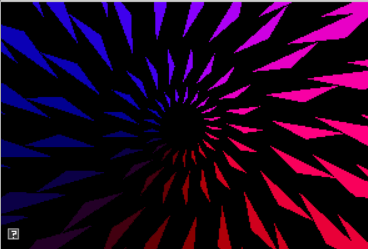## Assignment 4: You spin me round

In which, euler angles and rotation matrices become part of our 3D programming skillset

Due Friday, Oct 8, before midnight

The goals of this lab are to

• Understand the matrix form of euler angles

• Understand how to get euler angles from a rotations matrix

• Implement euler conversions

In this assignment, you will implement conversions between euler angles and matrices. To help test your conversions, you have been given a grid of spinning teapots as well as a console program for unit testing.

In the teapot viewer, you should see a grid of teapots. The UI on the top left allows you to enter test values. Each teapot tests a conversion function. This week, you will implement 6 of the teapots to match the top right. In your next assignment, you will implement the remaining two teapots.

 For this assignment, you do not need to implement the quaternion conversions that animate the bottom, left two teapots.
 To type a value into the GUI’s slider, do control+

## Get the source

On Github, do a `Fetch Upstream` to synchronize your forked repository with the class repository.

Then update the source on your local machine and rebuild.

``````> git pull
> cd build
> cmake ..; make``````

## 1. Euler to Matrix

In the file `libsrc/atkmath/matrix3-basecode.cpp`, implement the conversions from euler angles to a matrix.

 In this assignment, you will complete the implementations for Matrix3 in `libsrc/atkmath`. You do not need to modify `assignments/a4-euler/teapots.cpp` or `assignments/a4-euler/test_euler.cpp`.

To start, implement the function `Matrix3::fromEulerAnglesZYX()`. This function takes a parameter called angleRad which stores euler angles as a 3-tuple

 You will be implementing many similar functions. I recommend you add methods or functions to compute Rx, Ry, and Rz matrices.

In `matrix3.h`, you will see that `Matrix3` supports two types of syntax for accessing elements: indexing or by element name. For example,

``````std::cout << mM << std::endl; // indexing notation
std::cout << m11 << std::endl; // lecture matrix notation
mM[row][col] = 0.4;
m11 = 3;``````
• Implement `Matrix3::fromEulerAnglesZYX()`

• Implement `Matrix3::fromEulerAnglesXYZ()`

• Implement `Matrix3::fromEulerAnglesXZY()`

• Implement `Matrix3::fromEulerAnglesYZX()`

• Implement `Matrix3::fromEulerAnglesYXZ()`

• Implement `Matrix3::fromEulerAnglesZXY()`

Use the utility `test_euler.cpp` to help you implement your methods. Note that you can add more tests to this utility to help debug special cases!

``build> ../bin/a4-test-euler``

When a test fails, it prints the expected value followed by the computed value, for example,

``````ZYX
TEST FAIL
0 0 1
0 -1 0
1 0 0

1 0 0
0 1 0
0 0 1``````

## 2. Matrix to Euler

In class, we derived recipes for extract euler angles from XYZ and ZYX rotation matrices. In this question, derive the remaining formulas for extracting euler angles and implement them in your basecode.

In your implementation, be carefull of edge cases around 90 degrees!

• Implement `Matrix3::toEulerAnglesZYX()`

• Implement `Matrix3::toEulerAnglesXYZ()`

• Implement `Matrix3::toEulerAnglesXZY()`

• Implement `Matrix3::toEulerAnglesYZX()`

• Implement `Matrix3::toEulerAnglesYXZ()`

• Implement `Matrix3::toEulerAnglesZXY()`

To run the teapots from the build directory, type

``build> ../bin/a4-teapots``

To run the unit tests from the build directory, type

``build> ../bin/a4-test-euler``
 Implement the special cases (e.g. when the middle angle is 90 or -90 degrees) after implementing the main algorithms for each case.

## 3. Be Unique!

Implement your own unique animation or image in `a4-euler/unique.cpp`. Some ideas:

• Make a demo with rotating objects

• Make a cone that follows a trajectory

• Create a shape whose orientation and position is controlled using the keyboard### 4.1. What to hand-in

• Images, movies, gifs, as appropriate

### 4.2. How to hand-in

Check-in your code, images, and writeup and push the changes to Github. If everything is uploaded correctly, you will see your work on Github.

``````> cd animation-toolkit
> git commit -m "helpful message"
> git push``````

Best practice is to always commit changes as you work, rather than waiting until the end to commit changes. You can always revert to an old version if you need to!

### 4.3. Generating images, movies and gifs

Screenshots

On Ubuntu, you can take a screenshot by

• Prt Scrn to take a screenshot of the desktop.

• Alt + Prt Scrn to take a screenshot of a window.

Gifs

On Ubuntu, you can use Peek to create gifs.

Movies

On Ubuntu, you can use `recordmydesktop` to record movies in .ogv format.

By default, the whole desktop is recorded unless you give it a window id. To get the window id, call `xwininfo` and click on the window. Then pass the id to `recordmydesktop`.

``> recordmydesktop --windowid <WindowId> --no-sound  --v_quality 30 -o <name>.ogv``

To check the video, open it in firefox.

``> firefox <name>.ogv``
 Files larger than 100 MB cannot be checked into git. In general, videos should be less than 5 MB.

Update `assignments/a4-euler/Readme.md` to document the features in your assignment. Your readme should contain your images, gifs, and movies.
``![Alternate text here](/path/to/image.png)``